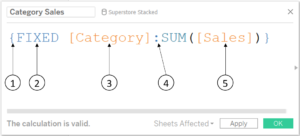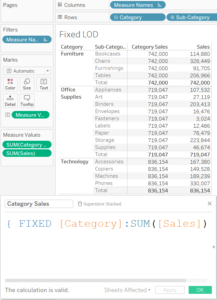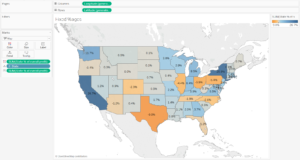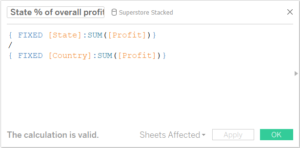## How to Talk 'Tablish': Lesson 2

by Joe Macari

Following on from my previous lesson, How to Talk Tablish: Lesson 1, we will be moving our attention away from table calculations and onto Level of Detail calculations (LODs). Que screams of sheer horror…Fear not dear reader, these are not as scary as you may initially think. You will soon be as fearless as a small dog chasing a bunch of pesky seals off a pier!

Prior to my time in Data School I had watched a former conference video with LODs and managed to replicate one of the charts without really knowing what was going on.

Having this Tablish translated into English really helped me get my head round them, and in some cases I find myself using these instead of table calculations (even when it is not necessary). Similar to the table calculations mentioned in Lesson 1 you just need to know the formula – here is an example where I want to see the total sales for each category:And here is the translation:

1. Note the curly bracket ‘{‘. When Tableau reads the curly bracket it goes ‘Ah, an LOD, this guy is cool’! In short – it’s what you need for an LOD.
2. You can choose one of 3 options in here (Fixed, Include or Exclude). For Fixed as used in the example above, we say “For Each [Category]”.
3. This is the field (or number of fields) you want to fix on.
4. The colon represents “give me“.
5. Anything after the colon is what is returned. In the case “the [Sum of Sales]”.

So over all we would say “For each [category] give me the [Sum of Sales]”.

And if you were to display the results of this in a table it would look a little something like this:We can see that the first measure values column (Category Sales) shows the total for the entire (or each) category at the sub-category level.

For the next example, lets look at something a little more practical and something that you might do in the real world rather than just for the sake of it. We want to show a map of each state and what proportion of the overall profit a state has contributed. Our final output will look like this:And the level of detail calculation used (State % of overall profit) looks like this:Here we use two separate Fixed LODs and we say:

For each [State], give me, the [Sum of profit]” and “For each [Country], give me the [Sum of profit]”.

So the LOD gives us the profit for each state and second gives us the total profit for the country. Dividing these gives us the proportion for each state to the overall country.

So there you have it, Lesson 2 of How to talk Tablish complete and you can now translate Fixed LOD calculations.

Remember, be the dog…

If you have any question or would like to leave any comments, please do so through my twitter page.Joe Macari

Mon 29 Jan 2018

Thu 25 Jan 2018

Tue 12 Dec 2017